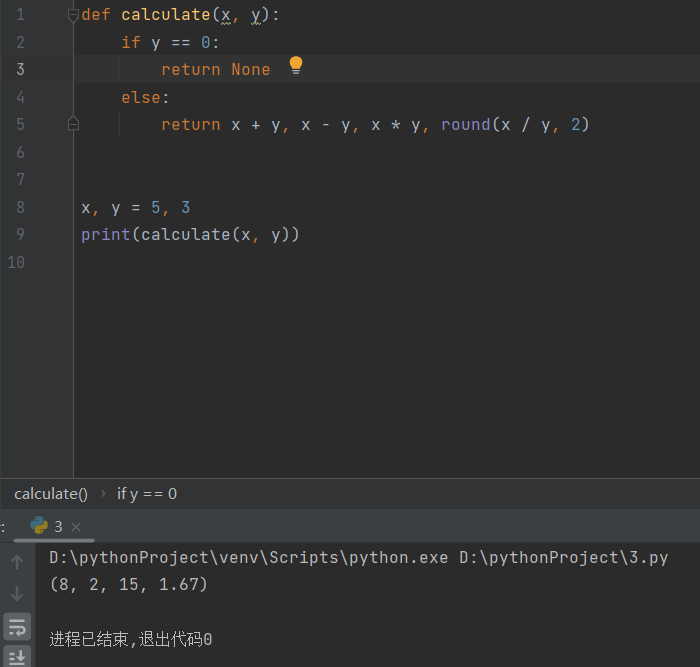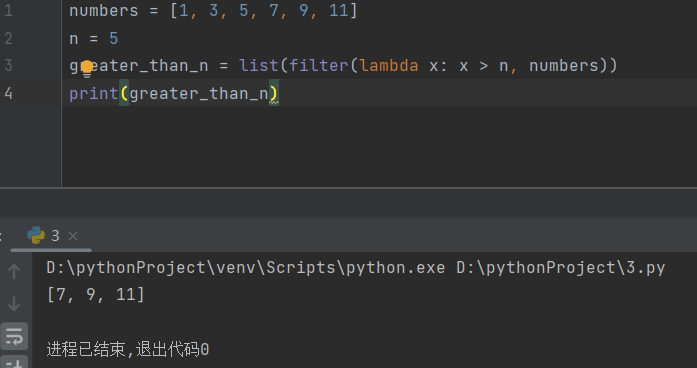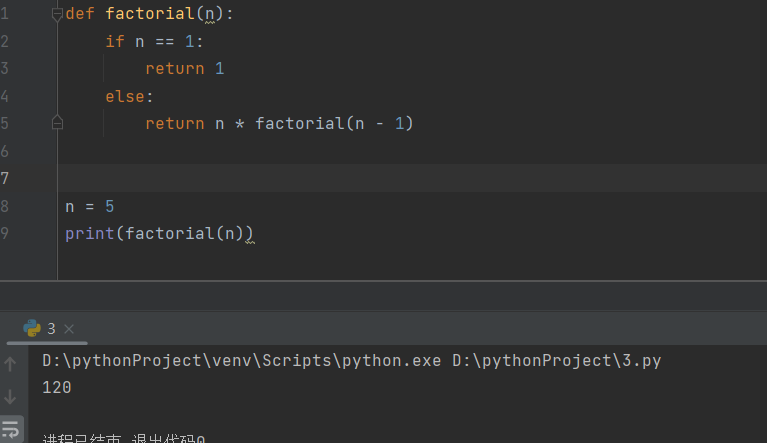# 作业要求：

1. 在自己的Python编程环境里，编程实现上述三道练习题，最好和参考答案代码不一样。

2. 测试运行你自己编写的代码和参考答案代码。

3. 如果测试运行时候，你的代码出现Bug，分析原因并调试代码。

4. 将上述1~3 要求写成博客发表。

## 一 、函数定义及使用

### 样例

``````def calculate(x, y):
if y == 0:
return None
else:
return x + y, x - y, x * y, round(x / y, 2)

x, y = 5, 3
print(calculate(x, y))``````## 二、Lambda函数

### 样例

``````numbers = [1, 3, 5, 7, 9, 11]
n = 5
greater_than_n = list(filter(lambda x: x > n, numbers))
print(greater_than_n)``````## 三、递归函数

### 样例

``````def factorial(n):
if n == 1:
return 1
else:
return n * factorial(n - 1)

n = 5
print(factorial(n))``````...全文
4 回复 打赏 收藏 转发到动态 举报【精品】15福师《面向web应用程序设计》在线作业两套[定义].pdf
【精品】15福师《面向web应用程序设计》在线作业两套[定义].pdf2023Python程序设计作业3： 函数应用2023Python程序设计作业5：Pandas基础技能及综合应用2023Python程序设计作业1： 基础语法之控制流程2023Python程序设计作业4：Numpy 和 Pillow在图像处理中的应用

159发帖与我相关我的任务
python 高校 江苏省·南通市• 近7日
• 近30日
• 至今